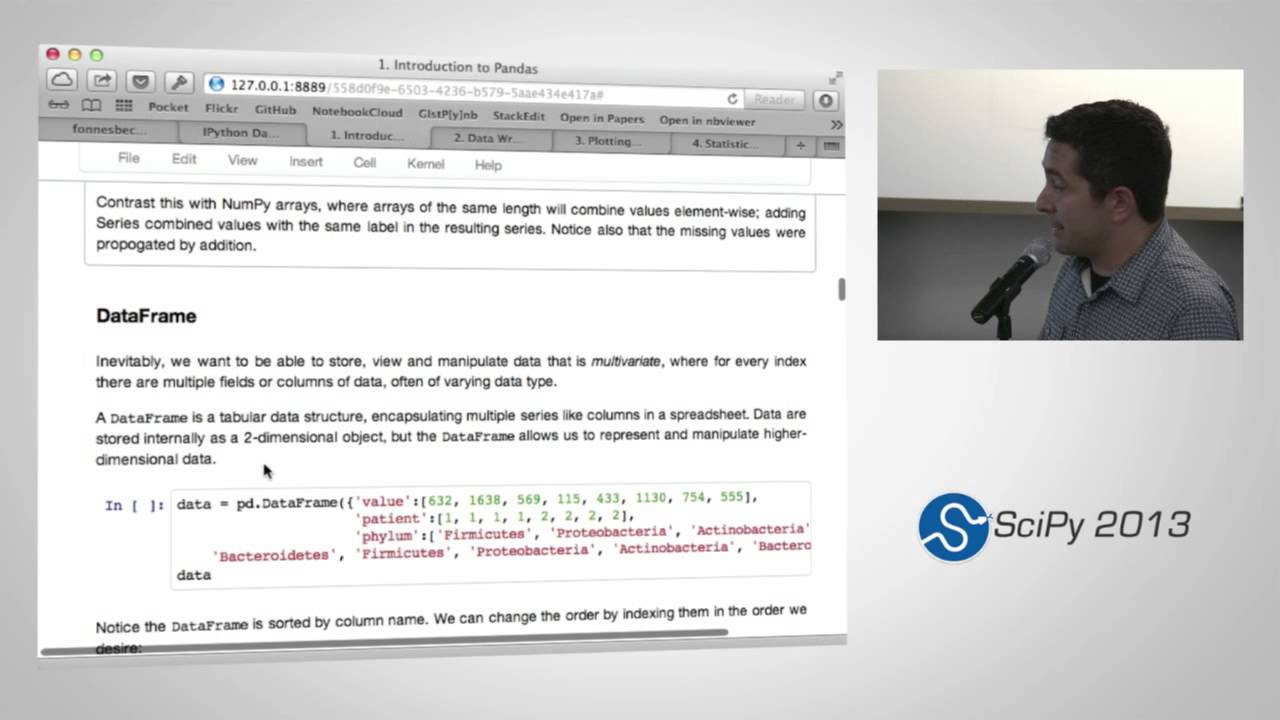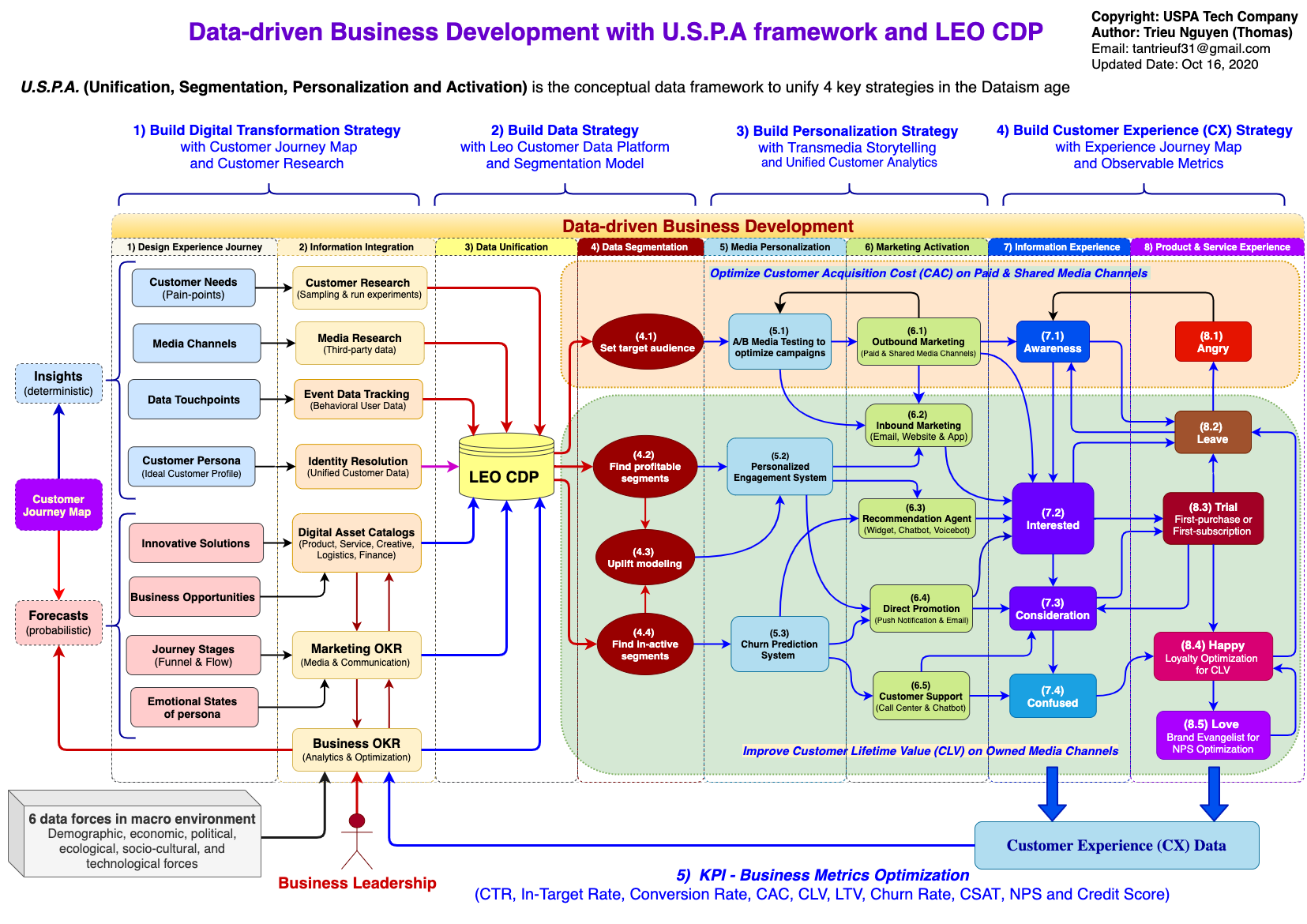## Monday, September 23, 2019

### Statistical Data Analysis in Python## Description

This tutorial will introduce the use of Python for statistical data analysis, using data stored as Pandas DataFrame objects. Much of the work involved in analyzing data resides in importing, cleaning and transforming data in preparation for analysis. Therefore, the first half of the course is comprised of a 2-part overview of basic and intermediate Pandas usage that will show how to effectively manipulate datasets in memory. This includes tasks like indexing, alignment, join/merge methods, date/time types, and handling of missing data. Next, we will cover plotting and visualization using Pandas and Matplotlib, focusing on creating effective visual representations of your data, while avoiding common pitfalls. Finally, participants will be introduced to methods for statistical data modeling using some of the advanced functions in Numpy, Scipy and Pandas. This will include fitting your data to probability distributions, estimating relationships among variables using linear and non-linear models, and a brief introduction to bootstrapping methods. Each section of the tutorial will involve hands-on manipulation and analysis of sample datasets, to be provided to attendees in advance.
The target audience for the tutorial includes all new Python users, though we recommend that users also attend the NumPy and IPython session in the introductory track.

## Outline

### Introduction to Pandas

• Importing data
• Series and DataFrame objects
• Indexing, data selection and subsetting
• Hierarchical indexing
• Sorting and ranking
• Missing data
• Data summarization

### Data Wrangling with Pandas

• Date/time types
• Merging and joining DataFrame objects
• Concatenation
• Reshaping DataFrame objects
• Pivoting
• Data transformation
• Permutation and sampling
• Data aggregation and GroupBy operations

### Plotting and Visualization

• Plotting in Pandas vs Matplotlib
• Bar plots
• Histograms
• Box plots
• Grouped plots
• Scatterplots
• Trellis plots

### Statistical Data Modeling

• Statistical modeling
• Fitting data to probability distributions
• Fitting regression models
• Model selection
• Bootstrapping

## Required Packages

• Python 2.7 or higher (including Python 3)
• pandas >= 0.11.1 and its dependencies
• NumPy >= 1.6.1
• matplotlib >= 1.0.0
• pytz
• IPython >= 0.12
• pyzmq